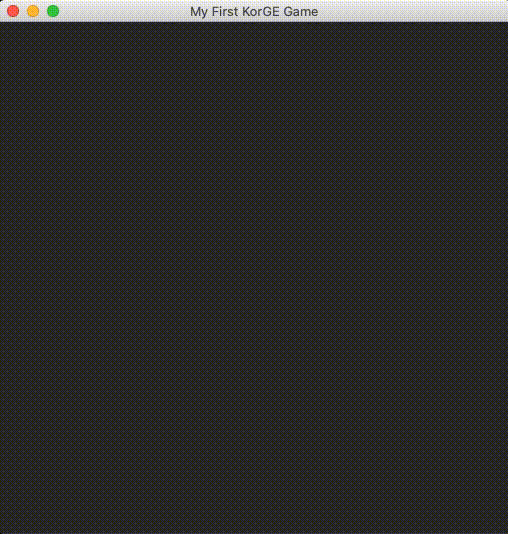## [Day08] 繼續學習動畫特效

Tweens

``````view.tween(view::x[10.0, 100.0], time = 1000.milliseconds)
``````

``````tapString.tween(tapString::alpha[0.0, 1.0], time = 1000.milliseconds)
``````

Animator

``````animate {
parallel {
tapString.moveToWithSpeed((image.scaledWidth) / 2 - (textString.count() * fontSize) / 4, (image.scaledHeight - image.scaledHeight / 4), 300.0, Easing.EASE_IN_OUT)
}
parallel{
tapString.alpha(0.0, time = 1000.milliseconds, easing = Easing.LINEAR)
if (tapString.alpha == 0.0) {
tapString.alpha = 1.0
}
}
}
``````